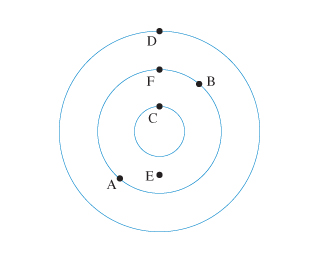# Problem: The figure shows the wavefronts of a circular wave.(1) What is the phase difference between points A and B?(2) What is the phase difference between points C and D?

###### FREE Expert Solution

The phase difference is the difference between the two successive crests and troughs.

87% (221 ratings)###### Problem Details

The figure shows the wavefronts of a circular wave.(1) What is the phase difference between points A and B?

(2) What is the phase difference between points C and D?